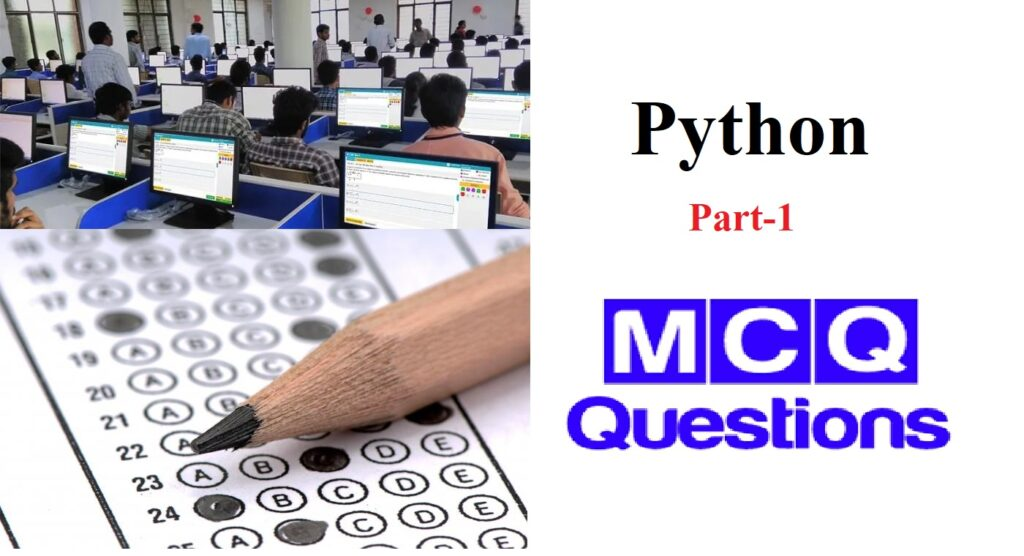# O Level Python MCQ-1Q 1 – What is a correct syntax to output “Hello World” in Python?

1. echo “Hello World”
2. p “Hello World”
3. print “Hello World”
4. echo “Hello World”

Answer : (C) print “Hello World”

Explanation : None

Q 2 – What is the maximum possible length of an identifier?

1. 16
2. 32
3. 64
4. None of these

Answer : (D) None of these

Explanation : The maximum possible length of an identifier is not defined in the python language. It can be of any number.

Q 3 – Who developed the Python language?

1. Zim Den
2. Guido van Rossum
3. Niene Stom
4. Wick van Rossum

Answer : (B) Guido van Rossum

Explanation : Python language was developed by Guido van Rossum in the Netherlands.

Q 4 – In which year was the Python language developed?

1. 1995
2. 1972
3. 1990
4. 1989

Explanation : None

Q 5 –  In which language is Python written?

1. Java
2. C
3. C++
4. .Net

Explanation : Python is written in C programming language, and it is also called CPython

Q 6 – What will be the output of the following code snippet?
print(2**3 + (5 + 6)**(1 + 1))

1. 129
2. 8
3. 121
4. None of these

Explanation : The above code will print 129 by following the BEDMAS rule of operator precedence.

Q 7 – What will be the datatype of the var in the below code snippet?
var = 10
print(type(var))
var = “Hello”
print(type(var))

1. str and int
2. int and int
3. str and str
4. int and str

Answer : (D) int and str

Explanation : None

Q 8 – How is a code block indicated in Python?

1. Brackets.
2. Indentation
3. Key
4. None of these

Explanation : None

Q 9 – What will be the output of the following code snippet?
a = [1, 2, 3]
a = tuple(a)
a = 2
print(a)

1. [2, 2, 3]
2. (2, 2, 3)
3. (1, 2, 3)
4. Error.

Explanation : Since we convert a to a tuple and then try to change its content, we will get an error since tuples are immutable.

Q 10 – What will be the output of the following code snippet?
print(type(5 / 2))
print(type(5 // 2))

1. float and int
2. int and float
3. float and float
4. int and int

Answer : (A) float and int

Explanation : None